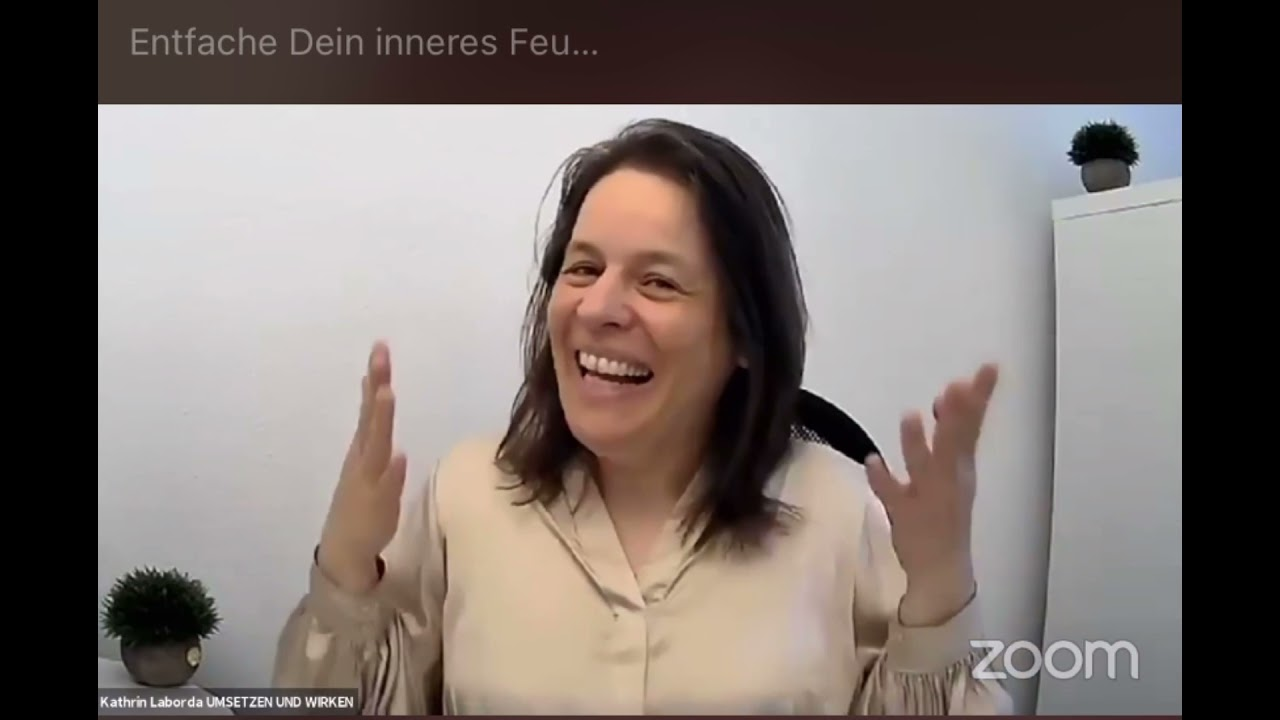# Sa05: Truss Analysis: Zero-Force Members

In this session we're going to talk about zero force members in trusses. What are they? Why do they come to exist? And how do we identify them?

What is a zero Force member when analyzing trusses we may come across members that carry no force. These are called zero force members. Why are the zero force members in trusses?

Naturally? One might question, the rationale for having such members in a structure. If a truss member does not carry any force. Why have it in the first place? There are two main reasons. For encountering zero force members in trusses one, a zero Force member could act as a bracing for preventing a force carrying compression member from buckling that is a zero Force member and stability to the structure to a force having a magnitude of zero could develop in a member at some point in time when the structure is subjected to a time-variant load let's examine. This scenario in more detail bridge structures are generally designed to withstand the load of moving vehicles where the load.

Position changes as a vehicle moves across the bridge. Suppose we have a truss bridge consisting of five joints along its bottom cord as a vehicle starts traveling on the bridge, the applied load position changes at time. T1. The load is distributed between joints 1 & 2 at time. T2. The load is distributed between joints 2, & 3 at t3.

The load is applied to joints 3 &, 4 &. So on this moving load pattern continues until the vehicle leaves the bridge, the bridge truss must be analyzed under each loading. Case notes that a truss member that actually carries an axial force in one loading case may become a zero Force member in another loading case. For example, when the load is applied at joints 1, & 2, the vertical member attached to joint 4 carries no internal force when the applied load moves to joints, 3 & 4, the vertical member attached to joint 2 becomes a zero Force member, the ability to identify zero force members in trusses is important for two reasons.

Once it speeds up the overall analysis of the. Truss - it helps to develop a better understanding of how internal forces are distributed in a structure. How do we identify zero force members in a truss? There are three basic rules for identifying zero force members without resorting to any calculations either trust joint is not subjected to any applied loads and is not supported by a pin or roller. Then the following rules can be applied repeatedly to identify all the zero force members. Rule 1 if the joint connects to members only where the. Angle between the members is different from 180 degrees.

Both members are zero force members. If this structure joint F connects to members only there is no load or support at the joint. Therefore, both EF and C F are zero force members rule two. If the joint connects two members only and the members are collinear that is the angle between them is 180 degrees. And one of the members carries no internal force. The other one is also a zero Force member. Consider the two members, a b and b c. If the force in.

Member a B is zero since the members are collinear bc, is also a zero Force member, Rule three joint connects three members only and two of the members are collinear. Then the third one is a zero Force member here joint D connects three members. Two of the members are collinear. Therefore, C D is a zero Force member. Let's.

Look at a few examples you here. Rule 1 applies to join D, therefore CD and DE are zero force members. You also Rule three applies to joint h. Hence, BH is a zero Force member you if we.

Consider P to be an axial force in a member vertically attached to joint D. Then according to rule three EF is a zero Force member you similarly, if we assume G X is the force in an actual member horizontally attached to joint G. Then according to rule 3, a G is a zero Force member you to determine the force in each of the remaining members, the simplified trust can be analyzed using the method of joints you in this example, rule 3 applies to join c, b, e and d in that sequence, therefore bc, b. E, DE and. Dg are zero force members. See if you can identify the zero force members in these trusses you.

18-Apr-2022

#### Leave Your Comment##### La Mejor Configuracion De Daltonismo Para Fortnite##### Two Way Classification In Tamil##### How To: Bypass Any Internet Filters Without A Proxy##### Smith & Archer Face Off At Lords As Australia Aim For Ashes Win!##### „Hörst Du Mich? Ich Steh‘ Auf Dich.“